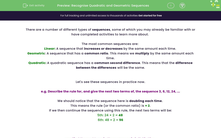# Recognise Quadratic and Geometric Sequences

In this worksheet, students will recognise all three types of sequences (linear, geometric and quadratic), find the rules which govern them, and apply these to find missing terms.Key stage:  KS 4

Year:  GCSE

GCSE Subjects:   Maths

GCSE Boards:   AQA, Eduqas, OCR, Pearson Edexcel,

Curriculum topic:   Algebra

Curriculum subtopic:   Sequences

Difficulty level:#### Worksheet Overview

There are a number of different types of sequences, some of which you may already be familiar with or have completed activities to learn more about.

The most common sequences are:

Linear: A sequence that increases or decreases by the same amount each time.

Geometric: A sequence that has a common ratio. This means we multiply by the same amount each time.

Quadratic: A quadratic sequence has a common second difference. This means that the difference between the differences will be the same.

Let's see these sequences in practice now.

e.g. Describe the rule for, and give the next two terms of, the sequence 3, 6, 12, 24, ...

We should notice that the sequence here is doubling each time

This means the rule (or the common ratio) is × 2.

If we then continue the sequence using this rule, the next two terms will be:

5th: 24 × 2 = 48

6th: 48 × 2 = 96

e.g. Find the next term in the sequence 3, 6, 11, 18, 27, ...

We first have to identify the sequence type here.

It isn't going up or down by the same amount so it can't be linear, but it also isn't multiplying each time, so it can't be a geometric sequence either.

This means it must be a quadratic sequence.

Step 1: Find the difference between the terms.

The difference between the first ans second terms is 3.

The difference between the second and third terms is 5.

The difference between the third and fourth terms is 7.

The difference between the fourth and fifth terms is 9.

Do you see how the difference is going up by 2 each time?

This means that the difference between the fifth and sixth terms is 11.

If we add this to the fifth term, we will find our next term:

27 + 11 = 38

In this activity, we will need to recognise all three types of sequences, find the rules which govern them, and apply these to find missing terms.

You should not use a calculator in this activity, but instead practise your mental arithmetic.

### What is EdPlace?

We're your National Curriculum aligned online education content provider helping each child succeed in English, maths and science from year 1 to GCSE. With an EdPlace account you’ll be able to track and measure progress, helping each child achieve their best. We build confidence and attainment by personalising each child’s learning at a level that suits them.

Get started